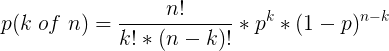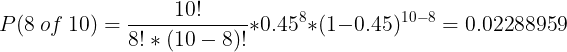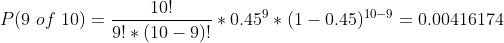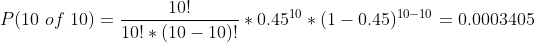Question

# Suppose that a university student has to take 10 independent courses in the final year. The class of honors awarded is based on the results of the final year examination. If a student gets A‐ or above for at least 8 courses, he/she will be awarded a first

Suppose that a university student has to take 10 independent courses in the final year. The class of honors awarded is based on the results of the final year examination. If a student gets A‐ or above for at least 8 courses, he/she will be awarded a first class honors.

Under ordinary circumstances, John will get A‐ or above for at least 8 courses with probability of 0.2. However, with a probability of 0.3, John will be in a low mood during the examination period. Under that low mood case, John will get A‐ or above for each course with probability 0.45.

(a) What is the probability that John will get A‐ or above for at least 8 courses given that he is in low mood during the examination period?

(b) What is the probability that John will achieve a first class honors?

a)

A distribution is a binomial distribution when

1) All trials are identical and independent.

2) two outcomes for each trial: Success, failure

3) Number of trials is fixed.

Here we have 10 courses. Each course is identical and independent. For each course, we have two outcomes: A- or above, below A-.

For low mood condition probability, John will get A‐ or above for each course is 0.45.

So This distribution is binomial with n=10 and p=0.45

Here at least 8 A- or above means we get A- or above in 8 or 9 or 10 courses.

So P(at least 8 A-)= P(8 of 10)+P(9 of 10)+P(10 of 10)

for a binomial distribution with n independent trials with probability p, equation to find the probability of k success in n trials isUsing this ideaP(at least 8 A-)=0.02288959+0.00416174+0.0003405=0.02739183

(round according to question)

P(at least 8 A- | low mood)=0.02739183

b)

For two events A and B we have P(A and B)=P(A)* P(B)

Here we need to find P(John achieves first-class).

This will be honored if and only if he gets at least 8 A-.

We have two conditions here: ordinary and low mood.

P(low mood)=0.3

Hence P(ordinary)= 1-0.3=0.7

In both situations, we have a chance for getting a first-class. So the sum of getting At least 8 A- in ordinary and low mood situations gives the probability of getting honored by first class. So

P(John achieves first-class)= P(ordinary and at least 8A-)+P(low mood and at least 8A-)

Ordinary

Here probability of at least 8 A- is 0.2. So P(at least 8 A- | ordinary)= 0.2

Then P(ordinary and at least 8A-)= P(at least 8 A- | ordinary) *P(ordinary)= 0.2*0.7=0.14

Low mood

We founded P(at least 8 A- | low mood)=0.02739183

So

P(low mood and at least 8A-)= P(at least 8 A- | low mood) *P(low mood)=0.02739183*0.3=0.008217549

So

P(first class)= 0.14+0.008217549= 0.148217549

#### Earn Coins

Coins can be redeemed for fabulous gifts.

Similar Homework Help Questions
• ### Comprehensive Income Tax Course: Module 1 4. Randy turned 16 last year and had his first...

Comprehensive Income Tax Course: Module 1 4. Randy turned 16 last year and had his first summer job. Even though his parents are claiming him as a dependent he wants to file a return in order to get his refund. He receives his W-2 and decides he can do his own return using form 1040-EZ. Which of the following information is not found on a Form W-2? a) The taxpayer’s Social Security number b) The taxpayer’s wages, tips and other...

• ### And there was a buy-sell arrangement which laid out the conditions under which either shareholder could...And there was a buy-sell arrangement which laid out the conditions under which either shareholder could buy out the other. Paul knew that this offer would strengthen his financial picture…but did he really want a partner?It was going to be a long night. read the case study above and answer this question what would you do if you were Paul with regards to financing, and why? ntroductloh Paul McTaggart sat at his desk. Behind him, the computer screen flickered with...